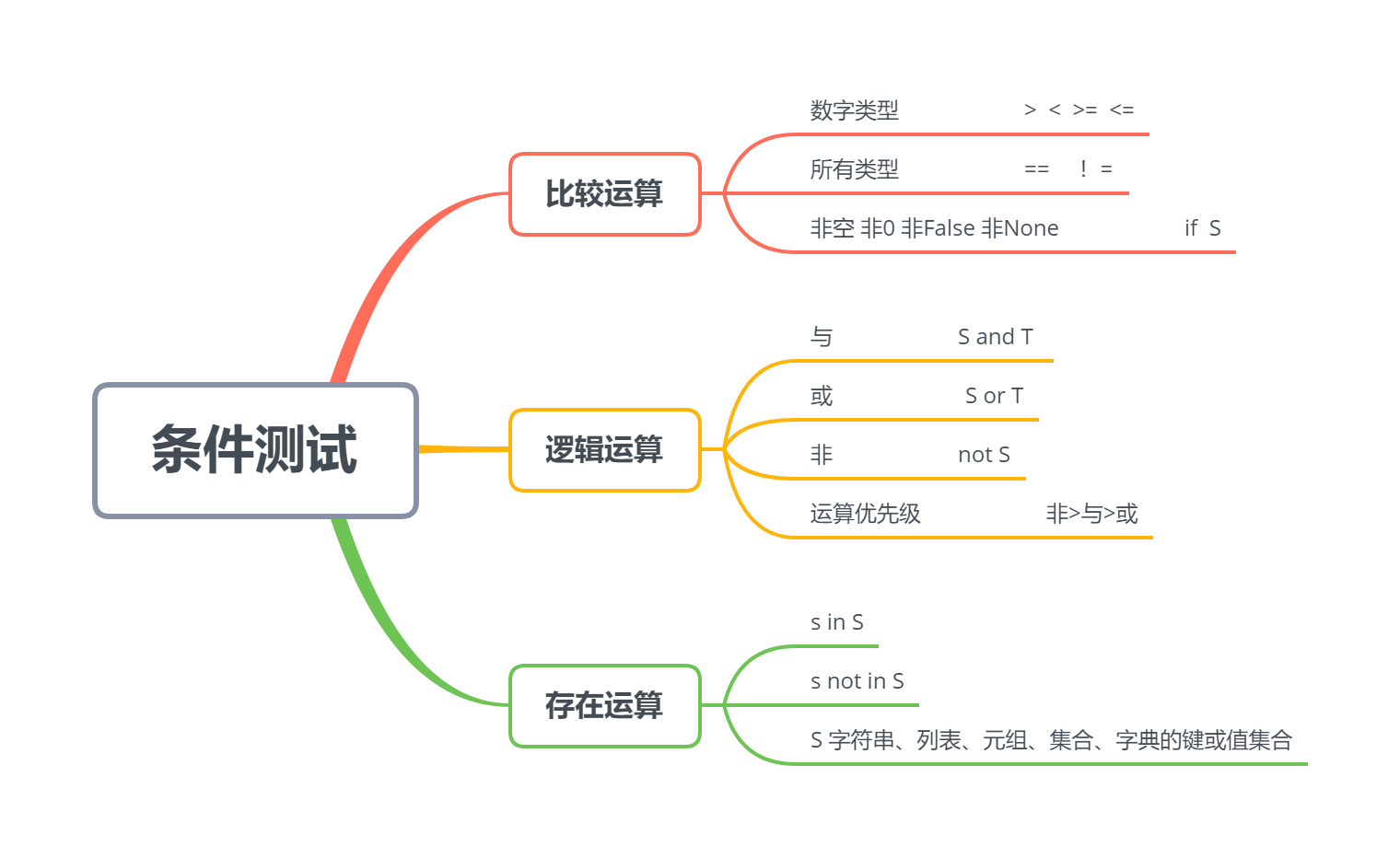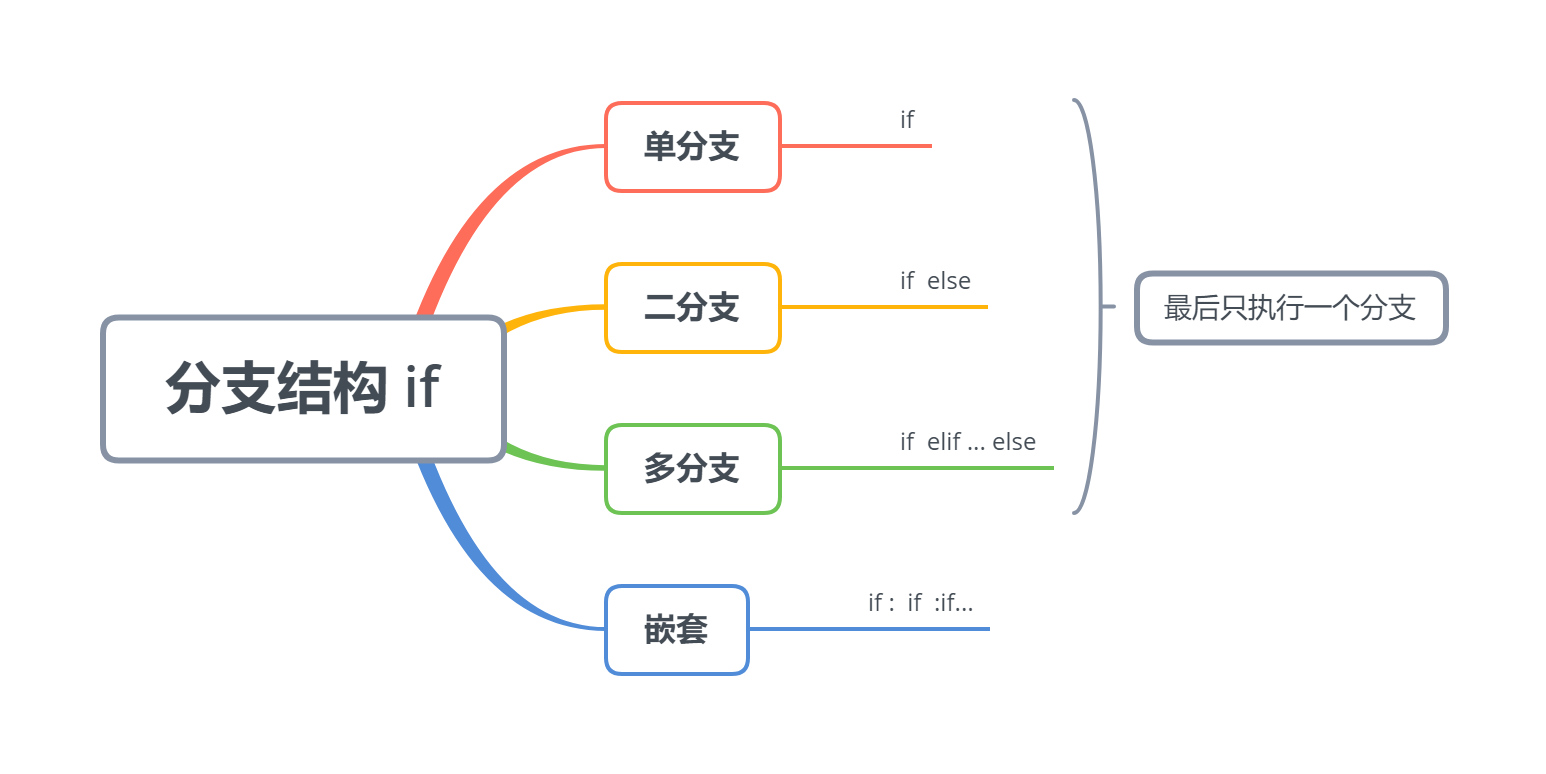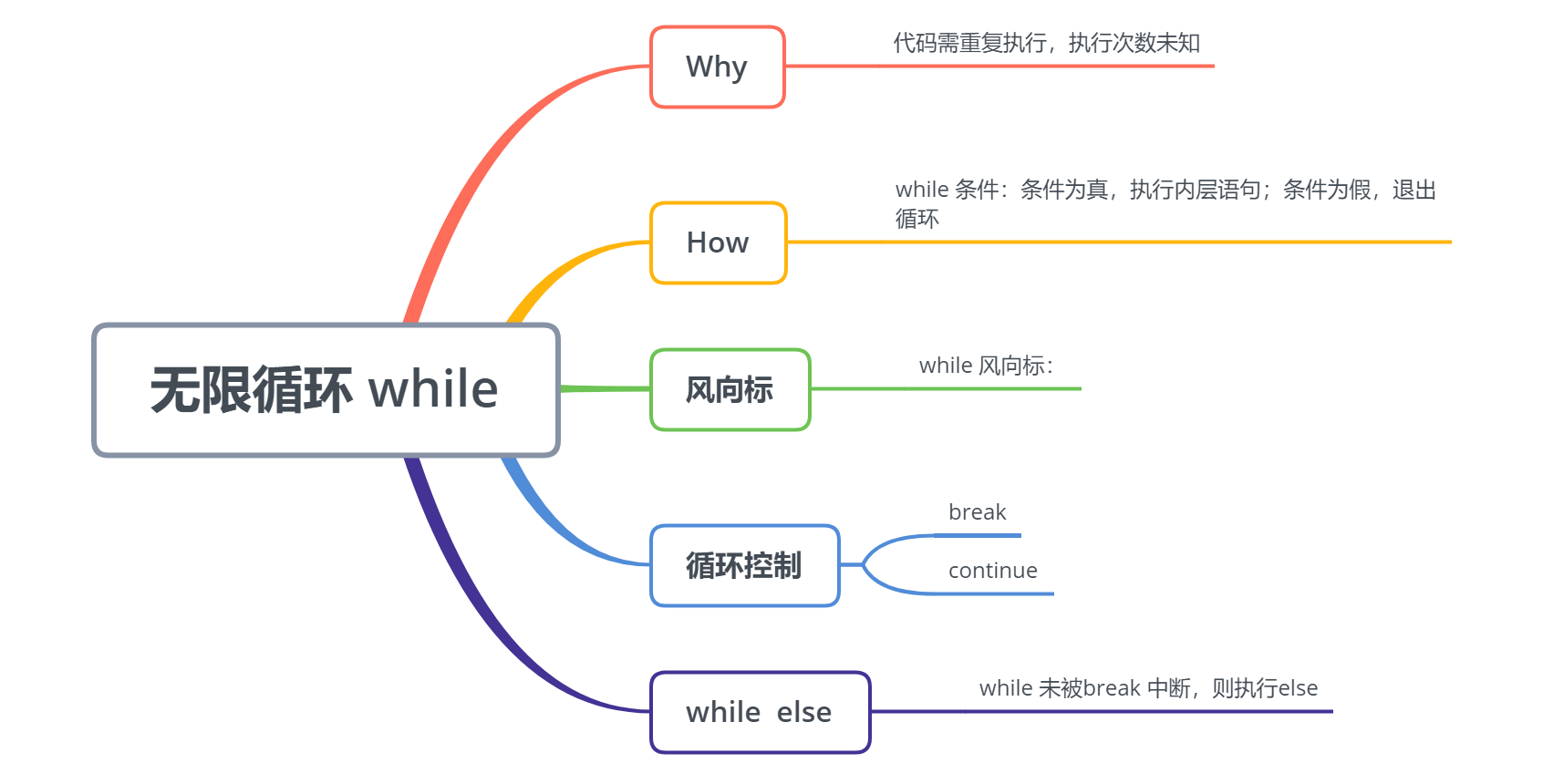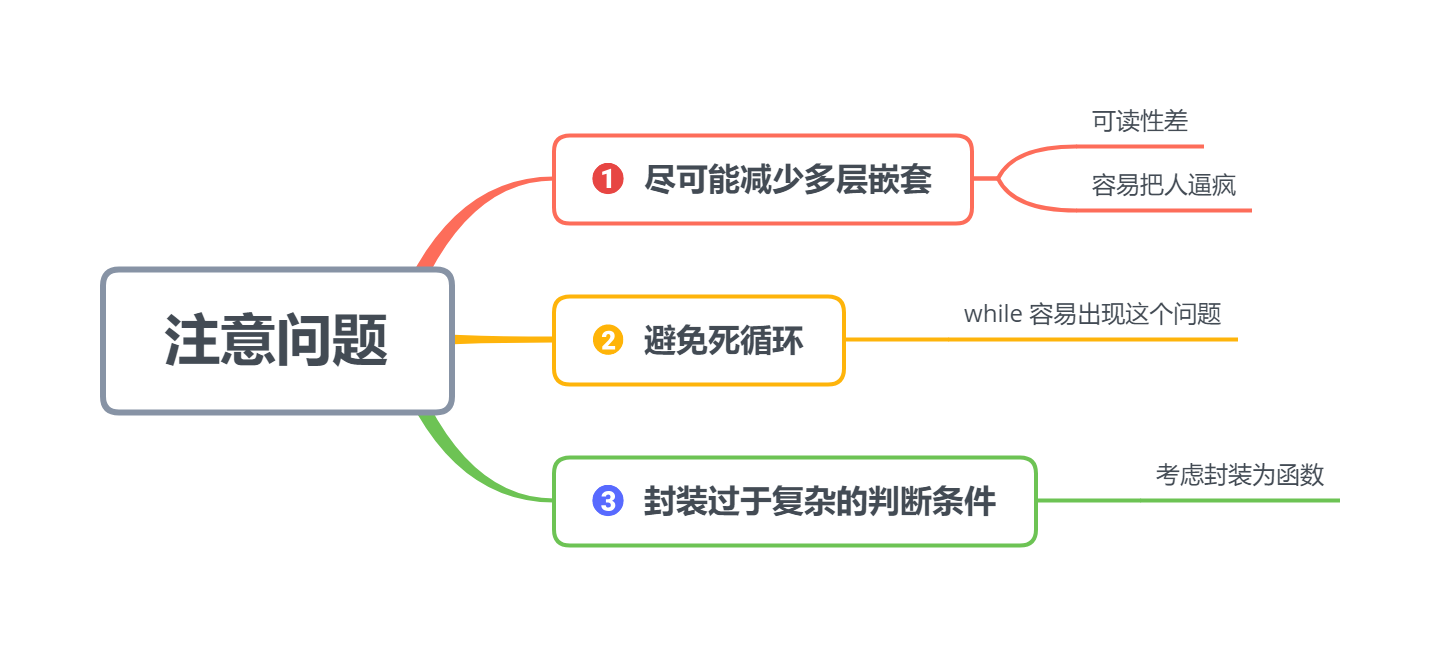# Python 基础（四） | 程序控制结构

• 2022 年 9 月 14 日
山东
• 本文字数：3782 字

阅读完需：约 12 分钟

# 第四章 程序控制结构

⭐本专栏旨在对 Python 的基础语法进行详解，精炼地总结语法中的重点，详解难点，面向零基础及入门的学习者，通过专栏的学习可以熟练掌握 python 编程，同时为后续的数据分析，机器学习及深度学习的代码能力打下坚实的基础。

🔥本文已收录于 Python 基础系列专栏： Python基础系列教程 欢迎订阅，持续更新。## 4.1 条件测试### 4.1.1 比较运算

a = 10b = 8print(a > b)     # 大于print(a < b)     # 小于print(a >= b)    # 大于等于print(a <= b)    # 小于等于print(a == b)    # 等于print(a != b)    # 不等于

TrueFalseTrueFalseFalseTrue

• 非空

ls = if ls:            # 数据结构不为空、变量不为0、None、False 则条件成立    print("非空")else:    print("空的")

非空

### 4.1.2 逻辑运算

• 与、或、非

a = 10b = 8c = 12print((a > b) and (b > c))    # 与print((a > b) or (b > c))     # 或print(not(a > b))             # 非

FalseTrueFalse

• 复合逻辑运算的优先级

• 非 > 与 > 或

print(True or True and False)

True

print((True or True) and False)

False

### 4.1.3 存在运算

cars = ["BYD", "BMW", "AUDI", "TOYOTA"]

print("BMW" in cars)print("BENZ" in cars)

TrueFalse

print("BMW" not in cars)print("BENZ" not in cars)

FalseTrue

## 4.2 分支结构——if 语句### 4.2.1 单分支

if 条件：

缩进的代码块

age = 8if age > 7:    print("孩子，你该上学啦！")

孩子，你该上学啦！

### 4.2.2 二分支

if 条件：

缩进的代码块

else：

缩进的代码块

age = 6if age > 7:    print("孩子，你该上学啦！")else:    print("再玩两年泥巴！")

再玩两年泥巴！

### 4.2.3 多分支

if 条件：

缩进的代码块

elif 条件：

缩进的代码块

elif 条件：

缩进的代码块

...

else：

缩进的代码块

age = 38if age < 7:    print("再玩两年泥巴")elif age < 13:    print("孩子，你该上小学啦")elif age < 16:    print("孩子，你该上初中了")elif age < 19:    print("孩子，你该上高中了")elif age < 23:    print("大学生活快乐")elif age < 60:    print("辛苦了，各行各业的工作者们")else:     # 有时为了清楚，也可以写成elif age >= 60:    print("享受退休生活吧") 

辛苦了，各行各业的工作者们

### 4.2.4 嵌套语句

age = eval(input("请输入年龄"))if age > 18:    is_public_place = bool(eval(input("公共场合请输入1，非公共场合请输入0")))    print(is_public_place)    if not is_public_place:        print("可以抽烟")    else:        print("禁止抽烟")        else:    print("禁止抽烟")            

请输入年龄19公共场合请输入1，非公共场合请输入01True禁止抽烟

## 4.3 遍历循环——for 循环### 4.3.1 主要形式

• for 元素 in 可迭代对象：

•   执行语句

### 4.3.2 执行过程

• 从可迭代对象中，依次取出每一个元素，并进行相应的操作

1、直接迭代——列表[ ]、元组( )、集合{ }、字符串" "

graduates = ("李雷", "韩梅梅", "Jim")for graduate in graduates:    print("Congratulations, "+graduate)

Congratulations, 李雷Congratulations, 韩梅梅Congratulations, Jim

2、变换迭代——字典

students = {201901: '小明', 201902: '小红', 201903: '小强'}for k, v in students.items():    print(k, v)for student in students.keys():#for student in students 同样是迭代键key    print(student)  

201901 小明201902 小红201903 小强201901201902201903

3、range()对象

res=[]for i in range(10000):    res.append(i**2)print(res[:5])print(res[-1])

[0, 1, 4, 9, 16]99980001

res = []for i in range(1, 10, 2):    res.append(i ** 2)print(res)

[1, 9, 25, 49, 81]

### 4.3.3 循环控制：break 和 continue

• break 结束整个循环

product_scores = [89, 90, 99, 70, 67, 78, 85, 92, 77, 82] # 1组10个产品的性能评分 # 如果低于75分的超过1个，则该组产品不合格i = 0for score in product_scores:    if score < 75:        i += 1     if i == 2:        print("产品抽检不合格")        break

产品抽检不合格

• continue 结束本次循环

product_scores = [89, 90, 99, 70, 67, 78, 85, 92, 77, 82] # 1组10个产品的性能评分 # 如果低于75分,输出警示print(len(product_scores))for i in range(len(product_scores)):    if product_scores[i] >= 75:        continue     print("第{0}个产品，分数为{1}，不合格".format(i, product_scores[i]))

10第3个产品，分数为70，不合格第4个产品，分数为67，不合格

### 4.3.4 for 与 else 的配合

product_scores = [89, 90, 99, 70, 67, 78, 85, 92, 77, 82] # 1组10个产品的性能评分 # 如果低于75分的超过1个，则该组产品不合格i = 0for score in product_scores:    if score < 75:        i+=1     if i == 2:        print("产品抽检不合格")        breakelse:    print("产品抽检合格")

产品抽检不合格

## 4.4 无限循环——while 循环### 4.4.1 为什么要用 while 循环

• 经典题目：猜数字

albert_age = 18#第1次guess = int(input(">>:"))if guess > albert_age :    print("猜的太大了")elif guess < albert_age :    print("猜的太小了")else:    print("猜对了")#第2次guess = int(input(">>:"))if guess > albert_age :    print("猜的太大了")elif guess < albert_age :    print("猜的太小了")else:    print("猜对了")

### 主要形式：

• while 判断条件：

•   执行语句

• 条件为真，执行语句

• 条件为假，while 循环结束

albert_age = 18 guess = int(input(">>:"))while guess != albert_age:     if guess > albert_age :        print("猜的太大了.")    elif guess < albert_age :        print("猜的太小了")    guess = int(input(">>:"))print("猜对了")

>>:20猜的太大了.>>:18猜对了

### 4.4.3 while 与风向标

albert_age = 18 flag = True     # 布尔类型while flag:    guess = int(input(">>:"))    if guess > albert_age :        print("猜的太大了")    elif guess < albert_age :        print("猜的太小了")    else:        print("猜对了")        flag = False

flag=True  while flag:      pass      while flag:          pass          while flag:                 flag=False     # 循环逐层判断，当flag为false时，循环会逐层退出

### 4.4.4 while 与循环控制 break、continue

albert_age = 18 while True:    guess = int(input(">>:"))    if guess > albert_age :        print("猜的太大了")    elif guess < albert_age :        print("猜的太小了")    else:        print("猜对了...")        break    # 当诉求得到满足，就跳出循环

i = 0while i < 10:    i += 1    if i % 2 == 0:        continue       # 跳出本次循环，进入下一次循环    print(i)

13579

### 4.4.5 while 与 else

count = 0while count <= 5 :    count += 1    print("Loop",count)else:    print("循环正常执行完啦")

Loop 1Loop 2Loop 3Loop 4Loop 5Loop 6循环正常执行完啦

### 4.4.6 再看两个例子

pets = ["dog", "cat", "dog", "pig", "goldfish", "rabbit", "cat"]

while "cat" in pets:    pets.remove("cat")pets

['dog', 'dog', 'pig', 'goldfish', 'rabbit']

not_read = ["红楼梦", "水浒传", "三国演义", "西游记"]have_read = []while not_read:     # not_read非空，循环继续，否则中止    book = not_read.pop()    have_read.append(book)    print("我已经读过《{}》了".format(book))print(not_read)print(have_read)

我已经读过《西游记》了我已经读过《三国演义》了我已经读过《水浒传》了我已经读过《红楼梦》了[]['西游记', '三国演义', '水浒传', '红楼梦']

# 4.5 控制语句注意问题### 4.5.1 尽可能减少多层嵌套

• 可读性差，容易把人搞疯掉

if 条件:    执行语句    if 条件：        执行语句        if...

### 4.5.2 避免死循环

# while True:    # print("欢迎订阅专栏")

### 4.5.3 封装过于复杂的判断条件

a, b, c, d, e = 10, 8, 6, 2, 0if (a > b) and (c >d) and (not e):    print("过于复杂")

numbers = (10, 8, 6, 2, 0)def judge(num):    a, b, c, d, e = num    x = a > b    y = c > d    z = not e    return x and y and zif judge(numbers):    print("简洁明了")## 评论